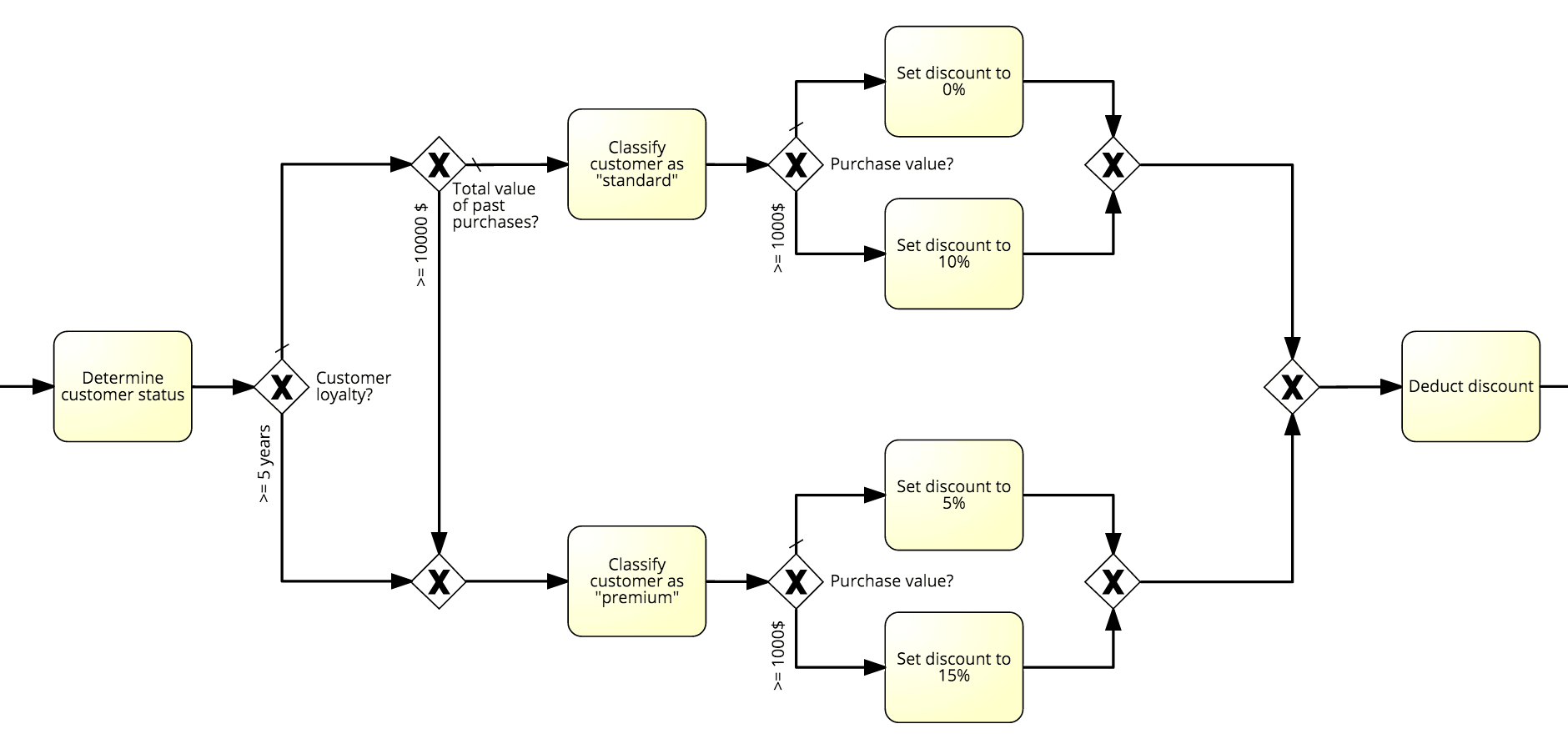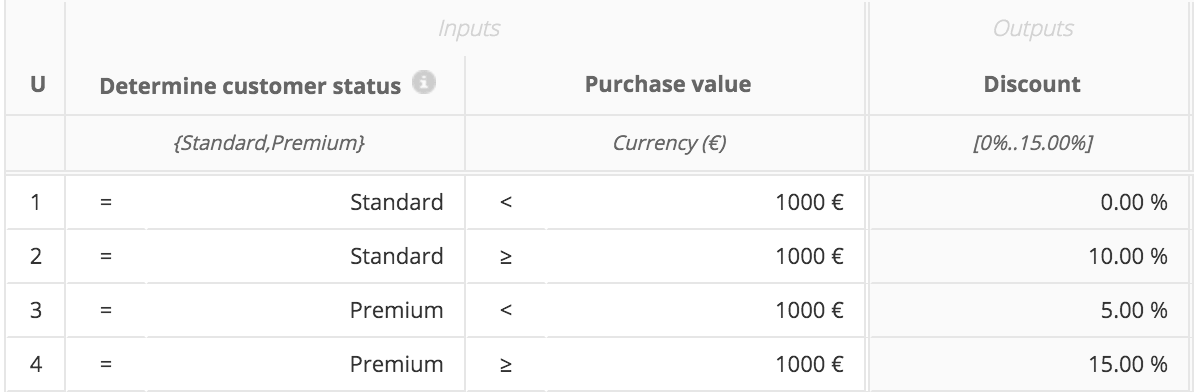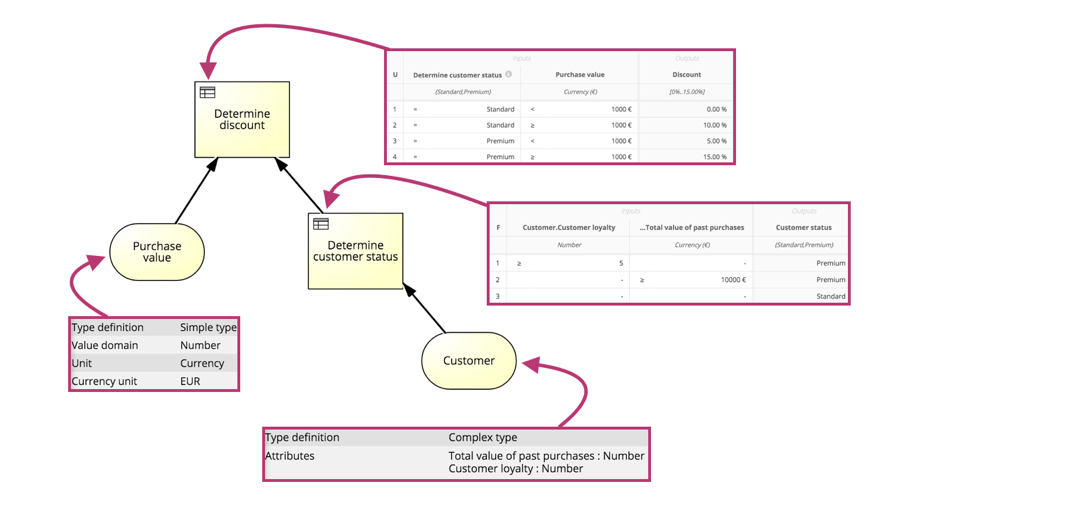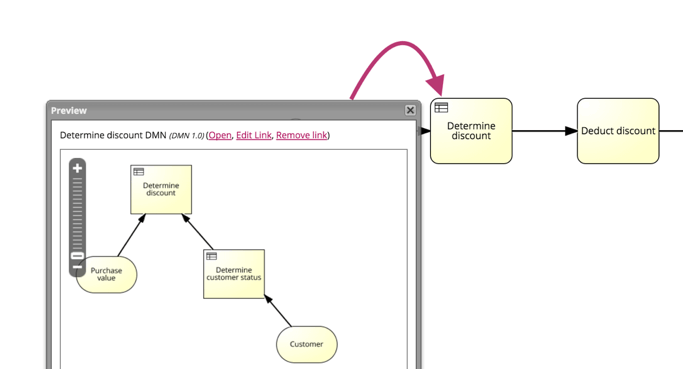As a BPMN modeler you are probably familiar with the following situation. You document a process that includes decision logic but even though the conditions are relatively straightforward you end up with an overly complex diagram.

As an example let’s have a look at a process that documents a product discount calculation. You want to determine the discount based on the parameters Customer Status and Purchase Value (in \$). The Customer Status is determined through Customer Loyalty (years as an active customer) and the Total Value of Past Purchases.As you can see, even this fairly simple decision adds tremendous complexity to the process model.

In a pure BPMN context you could thus make a trade-off between complexity and accuracy:

• Delete parts of the decision logic and document it, for example, in a text attribute.
• Refactor the decision logic into a sub process.

However both approaches are far from optimal.

If you move the decision logic to a sub-process you reduce the complexity of the original diagram significantly but the new model will still be hard to read and prone to inaccuracy. Changing details in the decision logic will often require extensive remodeling. If you move the decision logic into textual descriptions you end up with informal and often ambiguous descriptions that are hard to understand for your readers and impossible to process automatically.

The best solution for this problem is employing the Decision Model and Notation (DMN). DMN is an open and standardized modeling notation that is explicitly designed for modeling business decisions. In DMN decision logic isn’t represented as process flow but as a set of rules in a Decision Table. Each rule maps a set of input values to a set of outputs, for example, the Customer Status ‘Standard’ and a Purchase Value of less than 1000€ to a Discount of 0%.DMN diagrams can be seamlessly integrated into BPMN models.

Let’s take a look on how to refactor the decision logic of our example process into a DMN diagram.

First, we remodel the decision logic in DMN:

• We model input parameters – often represented in BPMN as Exclusive Gateways, for example, Purchase Value? - as Data Input elements.
• Decision logic is defined in the tables of Decision elements. Each rule in a decision table represents one Sequence Flow and its subsequent Task, which follow an Exclusive Gateway. For example '>= 1000\$?' and 'Set discount to 10%'.

To manage complexity we refactor several input parameters into one and split complex decisions into multiple sub decisions:Then we reference the DMN from a Business Rule Task in our BPMN diagram: